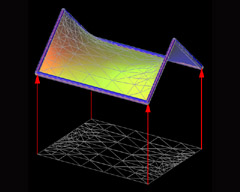# Touching Soap Films

An Introduction to Minimal Surfaces
By Hermann Karcher and Konrad Polthier

Up
Prev
Next

## Numerics and VisualizationFig 25. A numerical discretization of a minimal surface.

The numerical experiments on minimal surfaces have played a central part in the recent mathematical development and fruitfully complemented the theoretical research. In the initial phase most surfaces where numerically computed using elaborated methods for the analytic continuation of complex functions and integration of the Weierstraß representation formulas. Although the methods allowed to compute any surface given by its Weierstraß representation, this approach had the drawback that the Weierstraß formulas needed to be known in advance to do numerical experiments. Since existence of many surfaces is mathematically proved via the conjugate surface construction, e.g. most surfaces of Alan Schoen were initially proven this way, it was necessary to develop a numerical scheme for the conjugate surface construction.

In 1993, U. Pinkall and K. Polthier succeeded in the discovery of a numerical algorithm for the conjugate surface construction. Their method is based on a geometric discretization of minimal surfaces in triangle nets. Here the weighted edges emanating from each vertex of the discrete mesh must be balanced, leading to an explicit formula in terms of edge lengths and triangle angles. This geometric notion of the minimality condition was a key insight resulting in a powerful new numerical algorithm for the numerical computation of free boundary value problems for minimal surfaces via the conjugate surface construction.

Complementary to the numerical methods, the visualization of deforming surfaces was a major goal. The numerical methods use adaptive refinement of the surface triangulation generating a finer mesh at surface regions with high curvature and a coarser discretization at flat regions. During the deformation of minimal surfaces the curvature varies heavily causing the triangulation to vary too. It was a major challenge for visualization, solved by K. Polthier and M. Rumpf, to derive a method allowing discrete changes in the triangulation while ensuring a smooth perception to the observer during surface deformations.

The production of the video Touching Soap Films is a remarkable manifestation of the fruitful mutual enrichment of mathematical theory, applied numerics, and modern visualization techniques.